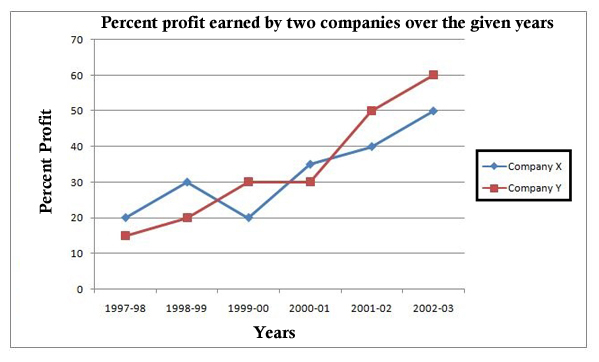## IBPS Quant Test 16

Instructions

Study the following graph to answer the given question.

% Profit=$$\frac{Income - Expenditure}{Expenditure}\times100$$Q 1

If the income of the company X in 1998-99 was equal to the expenditure of Company Y in 2001-02, what was the ratio of their respective profits ?

Q 2

For Company X, its income in 2001-02 was equal to its expenditure in 2002-03. What was the ratio of its respective incomes in these two years ?

Q 3

For Company Y, which year is the per cent of increase in per cent profit over that of previous year the highest ?

Q 4

In 1997-98, the expenditure of Company X was Rs. 40 crores. What was its income in that year ?

Q 5

What was the difference in expenditure of the two companies in 1990-00 ?